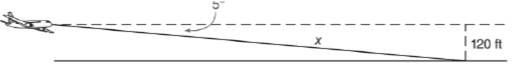Chapter 11.3, Problem 39EElementary Geometry For College St...

7th Edition
Alexander + 2 others
ISBN: 9781337614085

Solutions

Chapter
SectionElementary Geometry For College St...

7th Edition
Alexander + 2 others
ISBN: 9781337614085
Textbook Problem

In Exercises 39 to 45, angle measures should be given to the nearest degree; distance should be given to the nearest tenth of a unit.When her airplane is descending to land, the pilot notes an angle of depression of 5 ∘ . If the altimeter shows an altitude reading of 120 ft, what is the distance x from the plane to touchdown?To determine

To calculate:

The distance x from the plane to touchdown.

Explanation

Given:

When an airplane is descending to land, the pilot notes an angle of depression of 5. The altimeter shows an altitude reading of 120 ft.

Definition used:

The trigonometric ratio

 sinα=oppositehypotenuse

Calculation:

Consider the angle of depression as α.

Thus α=5.

From the figure, we see that sinα=120x.

sin5=120x

x=120sin5

Let us use Ti-83 calculator to find the value of x

Still sussing out bartleby?

Check out a sample textbook solution.

See a sample solution

The Solution to Your Study Problems

Bartleby provides explanations to thousands of textbook problems written by our experts, many with advanced degrees!

Get Started

In Problems 15-28, find the general solution to the given differential equation.

Mathematical Applications for the Management, Life, and Social Sciences

In Exercises 23-36, find the domain of the function. 25. f(x)=3x+1x2

Applied Calculus for the Managerial, Life, and Social Sciences: A Brief Approach

Evaluate the indefinite integral. sec2tan3d

Single Variable Calculus: Early Transcendentals, Volume I

The range of is: (−∞,∞) [0, ∞) (0, ∞) [1, ∞]

Study Guide for Stewart's Multivariable Calculus, 8th

The slope of the line tangent to the circle x2 + y2 = 100 at the point (6, 8) is: a) 34 b) 34 c) 43 d) 43

Study Guide for Stewart's Single Variable Calculus: Early Transcendentals, 8th